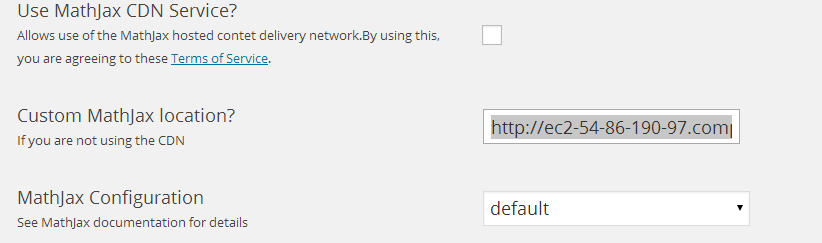This wordpress blog is configured to use the mathjax-latex plugin, along with a slightly customized MathJax configuration.  This allows inline latex equations to be specified with

$$...$$

and display latex to be specified with

$...$

or amsmath delimiters such as

\begin{equation}...\end{equation}

Here is a sample (Stokes theorem)

For blades $$F \in \bigwedge^{s}$$, and $$m$$ volume element $$d^k \mathbf{x}, s < k$$,
\begin{equation*}
\int_V d^k \mathbf{x} \cdot (\boldsymbol{\partial} \wedge F)
= \int_{\partial V} d^{k-1} \mathbf{x} \cdot F.
\end{equation*}

Here the volume integral is over a $$m$$ dimensional surface (manifold), $$\boldsymbol{\partial}$$ is the projection of the gradient onto the tangent space of the manifold, and $$\partial V$$ indicates integration over the boundary of $$V$$.

This was typeset with:

For blades $$F \in \bigwedge^{s}$$, and $$m$$ volume element $$d^k \mathbf{x}, s &lt; k$$,
\begin{equation*}
\int_V d^k \mathbf{x} \cdot (\boldsymbol{\partial} \wedge F)
= \int_{\partial V} d^{k-1} \mathbf{x} \cdot F.
\end{equation*}

Here the volume integral is over a $$m$$ dimensional surface (manifold), $$\boldsymbol{\partial}$$ is the projection of the gradient onto the tangent space of the manifold, and $$\partial V$$ indicates integration over the boundary of $$V$$.


My old (wordpress.com) hosted blog used the wp-latex plugin (implicitly), which is far inferior in many many ways (but is much faster to render):

• Any latex text had to be in one (sometimes very long) line, which was a maintainance pain.
• There was no support for equation numbering.  The mathjax-latex plugin (when the AMS numbering option is enabled) allows an author to use \label, and \eqref markers.
• mathjax-latex, if a custom copy of MathJax is used, can be configured to support \newcommand macros.  I can use a set of mathjax macros, for those places that I use peeters_macros.sty in standalone latex.  This allows me, for example, to write \Bx for $$\Bx$$ instead of \mathbf{x}, or \lr{…} for \left( … \right).  Because of these features I should be able to scrap my old latex-to-wordpress monstrosity of a script that I wrote to cater to the primitive subset of latex that wordpress.com allows by default.

### customizing MathJax

A customized version of MathJax requires laying down a copy of the distribution

cd /opt/bitnami/apps/wordpress/htdocs/wp-content/plugins/mathjax-latex
wget https://github.com/mathjax/MathJax/zipball/v2.3-latest
mv v2.3-latest mathjax-v2.3-latest.zip
unzip mathjax-v2.3-latest.zip
mv mathjax-MathJax-78ea6af MathJax


editing the configuration file (MathJax/config/default.js) as desired, and then pointing your wordpress plugin configuration at this modified configurationUnder the location you put a fully qualified URL for the MathJax.js in your unzipped distribution. For this site, after sftp copy of the MathJax directory to mathjax-latex, this was:

https://peeterjoot.com/wp-content/plugins/mathjax-latex/MathJax/MathJax.js

When trying this out on an amazon EC2 VM, this configuration step was easier than with godaddy, since godaddy only offers sftp access (on an EC2 image you can unzip on the VM directly which is much faster and easier).